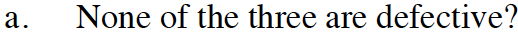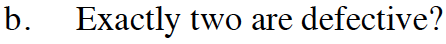Home > CCA2 > Chapter 12 > Lesson 12.1.2 > Problem12-37

12-37.
1. Three light bulbs are chosen at random from 15 bulbs, of which five are defective. What is the probability that:

1. None of the three are defective. Homework Help ✎

2. Exactly two are defective.Use combinations to solve this problem. For a review of combinations, see the Math Notes in Lesson 10.1.1.

$\text{Rember that probability = }\frac{ # \text{ of desirable outcomes}}{# \text{ of possible outcomes}}.$Use the same method as part (a).